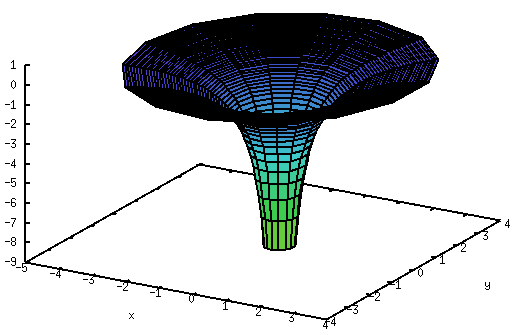There is something curious about gravity in general relativity. Specifically, the gravitational potential.

In high school, we were taught about this mysterious thing called “potential energy” or “gravitational potential”, but we were always assured that it’s really just the difference between potentials that matters. For instance, when you drop a stone from a tall tower, its final velocity (ignoring air resistance) is determined by the difference in gravitational potential energy at the top and at the bottom of the tower. If you study more sophisticated physics, you eventually learn that it’s not the gravitational potential, only its gradient that has physically observable meaning.

Things are different in general relativity. The geometry of spacetime, in particular the metric and its components are determined by the gravitational potential itself, not its gradient. In particular, we have

$$g_{00} = 1 – \frac{2GM}{c^2r}$$

in the infamous Schwarzschild metric, where $$g$$ is the metric tensor, $$G$$ is the universal gravitational constant, $$c$$ is the speed of light, $$M$$ is the mass of the gravitating object, and $$r$$ is the distance from it. Since the Newtonian gravitational field is given by $$U=-GM/r$$, this means

$$g_{00} = 1 – \frac{2}{c^2}U.$$

This quantity has physical significance. For instance, the angle by which light is deflected when it passes near a star is given by $$4c^{-2}U$$.

So then, what is the value of $$U$$ here on the surface of the Earth? Why, it’s easy. The mass of the Earth is $$M_E=6\times 10^{24}$$ kg, its radius is roughly $$R_E=6.37\times 10^6$$ m, so

$$\frac{1}{c^2}U_E=\frac{GM_E}{c^2R_E} \simeq 7\times 10^{-10}.$$

You could be forgiven for thinking that this is the right answer, but it really isn’t. For let’s just calculate the gravitational potential of the Sun as felt here on the Earth. Yes, I know, the Sun is quite a distance away and all, but play along, will you.

The mass of the Sun is $$M_\odot=2\times 10^{30}$$ kg, its distance from the Earth is $$R_\odot=1.5\times 10^{11}$$ m. So for the Sun,

$$\frac{1}{c^2}U_\odot=\frac{GM_\odot}{c^2R_\odot} \simeq 10^{-8}.$$

Whoops! This is more than an order of magnitude bigger than the Earth’s own gravitational potential! So right here, on the surface of the Earth, $$U$$ is dominated by the Sun!

Or is it? Let’s just quickly check what the gravitational potential of the Milky Way is here on the Earth. The Sun is zipping around the center in what we believe is a roughly circular orbit, at a speed of 250 km/s. We know that for a circular orbit, the velocity is $$v_\star=\sqrt{GM_\star/R_\star}=\sqrt{U_\star}$$, so

$$\frac{1}{c^2}U_\star = \frac{v_\star^2}{c^2} \simeq 7\times 10^{-7}.$$

This is almost two orders of magnitude bigger than the gravitational potential due to the Sun! So here, on the surface of the Earth, the gravitational potential is dominated by the large concentration of mass near the center of the Milky Way, some 8 kiloparsecs (25 thousand light years) from here. Wow!

But wait a minute, is this the end? There is the Local Supercluster of galaxies of which the Milky Way is part. Its mass $$M_V$$ is believed to be about $$10^{15}$$ times the mass of the Sun, and it is believed to be centered near the Virgo cluster, about 65 million light years or about $$R_V=6.5\times 10^{23}$$ meters away. So (this is necessarily a crude estimate, but it will serve as an order-of-magnitude value):

$$\frac{1}{c^2}U_V=\frac{GM_V}{c^2R_V} \simeq 2.3\times 10^{-6}.$$

This value for the gravitational potential right here on the Earth’s surface, astonishingly, is more than 3,000 times the gravitational potential due to the Earth’s own mass. Is this the end? Or would more distant objects exert an even greater influence on the gravitational field here on the Earth? The answer is the latter. That is because as we look further into the distant universe, the amount of mass we see goes up by the square of the distance, but their gravitational influence goes down by only the first power of the distance. So if you look at 10 times the distance, you will see 100 times as much matter; the gravitational influence of each unit of matter will decrease by a factor of 10 but overall, with a hundred times as much mass, the total gravitational influence will still go up tenfold.

So the local gravitational field is dominated by the most distant matter in the universe.

And by local gravitational field, I of course mean the local metric, which in turn determines how light is deflected, how clocks slow down, how the wavelength of photons shifts.

Insanely, we may not even know how fast a “true” clock in our universe runs, one that is free of gravitational influences, because we don’t know the actual magnitude of the sum total of all gravitational influences here on the Earth.

Gravity is weird.

### One Response to “Relativity and the gravitational potential”

1. […] have received a surprising number of comments to my recent post on the gravitational potential, including a criticism: namely that what I am saying is nonsense, […]